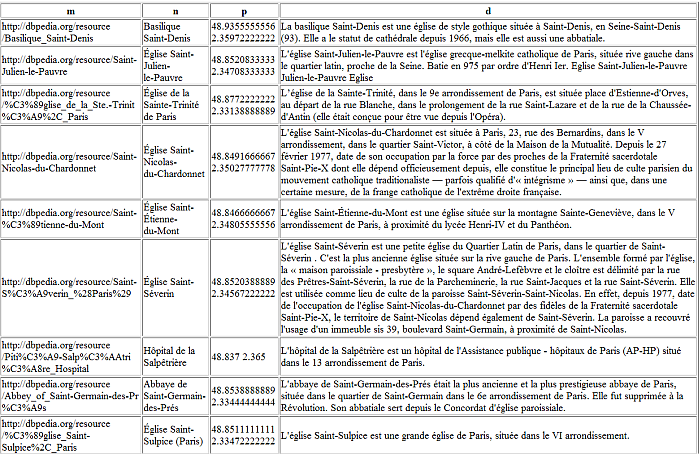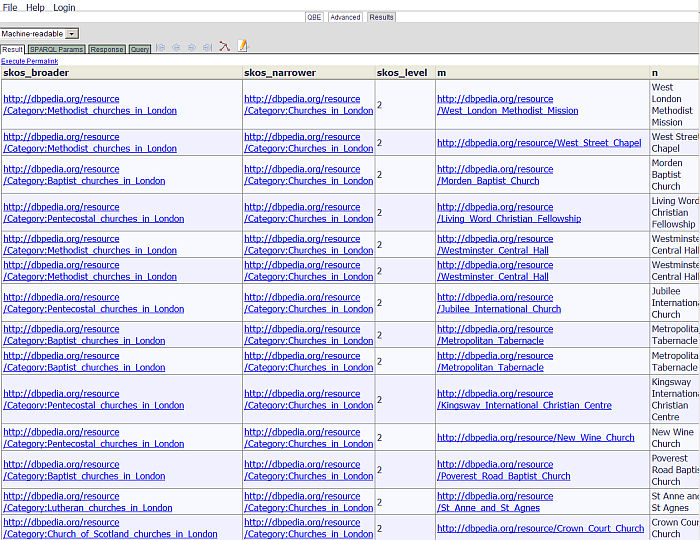### 16.2.11.Transitivity in SPARQL

Virtuoso SPARQL allows access to Virtuoso's SQL transitivity extension. Read the SQL section for a definition of the options.

The SPARQL syntax is slightly different from the SQL, although the option names and meanings are the same.

In SPARQL, the transitive options occur after a subquery enclosed in braces:

The below produces all the IRI's that are the same as <http://dbpedia.org/resource/New_York>.

```SPARQL
SELECT ?syn
WHERE
{
{
SELECT ?x ?syn
WHERE
{
{ ?x owl:sameAs ?syn }
UNION
{ ?syn owl:sameAs ?x }
}
}
OPTION ( TRANSITIVE, t_in (?x), t_out (?syn), t_distinct, t_min (0) )
FILTER (?x = <http://dbpedia.org/resource/New_York>) .
}
```

In this case, we provide a binding for ?x in the filter outside of the transitive subquery. The subquery therefore is made to run from in to out. The same effect would be accomplished if we bound ?syn and SELECT ?x, the designations of in and out are arbitrary and for transitive steps that can be evaluated equally well in both directions this makes no difference.

The transitive subquery in the above is

```{SELECT ?syn
WHERE
{
{ SELECT ?x ?syn
WHERE
{
{ ?x owl:sameAs ?syn }
UNION
{ ?syn owl:sameAs ?x}
}
} OPTION (TRANSITIVE, t_in (?x), t_out (?syn), t_distinct, t_min (0) )
}
} .
```

Leaving out the option would just look for one step of owl:sameAs. Making it transitive will apply the subquery to all bindings it produces until all are visited at least once (the t_distinct modifier).

If the transitive step consists of a single triple pattern, there is a shorthand:

```  <alice> foaf:knows ?friend option (transitive t_min (1))
```

will bind ?friend to all directly and indirectly found foaf:known individuals. If t_min had been 0, Malice> would have also been in the generated bindings.

The syntax is

```  option (transitive transitivity_option[,...])

transitivity_option ::=  t_in (<variable_list>)
| t_out (<variable_list>)
| t_distinct
| t_shortest_only
| t_no_cycles
| t_cycles_only
| t_min (INTNUM)
| t_max (INTNUM)
| t_end_flag (<variable>)
| t_step (<variiable_or_step>)
| t_direction INTNUM

variable_list ::= <variable> [,...]

variable_or_step ::= <variable> | path_id' | 'step_no'
```

Unlike SQL, variable names are used instead of column numbers. Otherwise all the options have the same meaning.

Some examples of the use of transitivity are:

#### Collection of Transitivity Option Demo Queries for SPARQL

##### Example for finding out what graphs contain owl:sameAs for "New York"

To find out what graphs contain owl:sameAs for Dan York, we do

```   SELECT ?g ?x count (*) as ?count
WHERE {
{
SELECT ?x ?alias ?g
WHERE {
{
GRAPH ?g {?x owl:sameAs ?alias }
}
UNION
{
GRAPH ?g {?alias owl:sameAs ?x}
}
}
}
OPTION ( TRANSITIVE,
t_in (?x),
t_out (?alias),
t_distinct,
t_min (1)) .
FILTER (?x = <http://dbpedia.org/resource/New_York> ) .
}
```

Here we select all paths that start with the initial URI and pass through one or more sameAs statements. Each step produces a result of the transitive subquery. The graph where the sameAs triple was found is returned and used as the grouping column. In this way we see how many times each graph is used. Note that graphs are counted many times since the graphs containing immediate sameAs statements are counted for paths of length 1, then again as steps on paths that reach to their aliases and so on.

##### Example for query that takes all the people known by Tim Berners-Lee, to a depth between 1 and 4 applications of the subquery

This query takes all the people known by kidehen, to a depth between 1 and 4 applications of the subquery. It then sorts them by the distance and the descending count of connections of each found connection. This is equivalent to the default connections list shown by LinkedIn.

```  SPARQL
SELECT ?o ?dist ((SELECT COUNT (*) WHERE {?o foaf:knows ?xx}))
WHERE
{
{
SELECT ?s ?o
WHERE
{
?s foaf:knows ?o
}
} OPTION ( TRANSITIVE,
t_distinct,
t_in(?s),
t_out(?o),
t_min (1),
t_max (4),
t_step ('step_no') as ?dist ) .
FILTER (?s= <http://www.w3.org/People/Berners-Lee/card#i>)
}
ORDER BY ?dist DESC 3
LIMIT 50
```
##### Example for query that takes all the people known by Tim Berners-Lee, to a depth between 2 and 4 applications of the subquery

This query takes all the people known by kidehen, to a depth between 2 and 4 applications of the subquery. It then sorts them by the distance and the descending count of connections of each found connection. This is equivalent to the default connections list shown by LinkedIn.

```  SPARQL
SELECT ?o ?dist ((SELECT COUNT (*) WHERE {?o foaf:knows ?xx}))
WHERE
{
{
SELECT ?s ?o
WHERE
{
?s foaf:knows ?o
}
} OPTION ( TRANSITIVE,
t_distinct,
t_in(?s),
t_out(?o),
t_min (2),
t_max (4),
t_step ('step_no') as ?dist) .
FILTER (?s= <http://www.w3.org/People/Berners-Lee/card#i>)
}
ORDER BY ?dist DESC 3
LIMIT 50
```
##### Example for finding how two people know each other and what graphs are involved in the connection

To find how two people know each other and what graphs are involved in the connection, we do:

```  SPARQL
WHERE
{
{
SELECT ?s ?o ?g
WHERE
{
graph ?g {?s foaf:knows ?o }
}
} OPTION ( TRANSITIVE,
t_distinct,
t_in(?s),
t_out(?o),
t_no_cycles,
T_shortest_only,
t_step ('path_id') as ?path,
t_step ('step_no') as ?step,
t_direction 3) .
FILTER (?s= <http://www.w3.org/People/Berners-Lee/card#i>
}
LIMIT 20
```

This query binds both the t_in and t_out variables. The ?g is left as a free variable. Also, specifying ?s and the system defined constants step_no and path_id as with t_step, we get for each transitive step a row of results with the intermediate binding of ?s, the count of steps from the initial ?s and a distinct identifier for the individual path, since there can be many distinct paths that link the ?s and ?o specified in the filter.

See the SQL transitive option section for details on the meaning of step_no and path_id.

##### Example for TBox Subsumption

Subsumption Demo Using Transitivity Clause

Yago Class Hierarchy (TBox) Subsumption

AlphaReceptors

```# all subjects with IRI: <http://dbpedia.org/class/yago/AlphaReceptor105609111>,
# that are sub-classes of anything (hence ?y)
# without restrictions on tree levels
SELECT ?y
FROM <http://dbpedia.org/resource/classes/yago#>
WHERE
{
{
SELECT *
WHERE
{
?x rdfs:subClassOf ?y .
}
}
OPTION (TRANSITIVE, t_distinct, t_in (?x), t_out (?y) ) .
FILTER (?x = <http://dbpedia.org/class/yago/AlphaReceptor105609111>)
}
```
##### Example for Receptors
```SELECT ?x
FROM <http://dbpedia.org/resource/classes/yago#>
WHERE
{
{
SELECT *
WHERE
{
?x rdfs:subClassOf ?y .
}
} OPTION (transitive, t_distinct, t_in (?x), t_out (?y) ) .
FILTER (?y = <http://dbpedia.org/class/yago/Receptor105608868>)
}
```
##### Inference Rule example using transitive properties from SKOS vocabulary

The following example demostrates the steps how to retrieve the skos ontology, add triples for skos:broaderTransitiveinto the graph, define inference rule, and at the and execute sparql query with inference rule and transitivity option. The queries were executed against the LOD instance (http://lod.openlinksw.com):

1. Make the Context graph, assuming you don't want to load entire SKOS vocabulary into our Quad Store:

```SQL>SPARQL
PREFIX skos: <http://www.w3.org/2004/02/skos/core#>
PREFIX rdfs: <http://www.w3.org/2000/01/rdf-schema#>
skos:narrower rdfs:subPropertyOf skos:narrowerTransitive };
```
2. OR Load entire SKOS ontology into Quad Store via iSQL interface (commandline or HTML based Conductor):

```SQL>DB.DBA.RDF_LOAD_RDFXML (http_get ('http://www.w3.org/2009/08/skos-reference/skos-owl1-dl.rdf'), 'no', 'urn:rules.skos');
Done.
```
3. Make Context Rule:

```SQL>rdfs_rule_set ('skos-trans', 'urn:rules.skos');
Done.
```
4. Go to SPARQL endpoint, for ex. http://lod.openlinksw.com/sparql

5. Use inference rule pragma to set context rule for SPARQL query, i.e:

```SPARQL
DEFINE input:inference "skos-trans"
PREFIX p: <http://dbpedia.org/property/>
PREFIX dbpedia: <http://dbpedia.org/resource/>
PREFIX category: <http://dbpedia.org/resource/Category:>
PREFIX rdfs: <http://www.w3.org/2000/01/rdf-schema#>
PREFIX skos: <http://www.w3.org/2004/02/skos/core#>

SELECT DISTINCT ?m ?n ?p ?d
WHERE
{
?m rdfs:label ?n.
?m skos:subject ?c.
?c skos:broaderTransitive category:Churches_in_Paris OPTION (TRANSITIVE) .
?m p:abstract ?d.
?m geo:point ?p
FILTER ( lang(?n) = "fr" )
FILTER ( lang(?d) = "fr" )
}
```
6. You will get 22 rows returned from the query. Note that for comparison, if the option (transitive) is ommitted, then only 2 rows will be returned in our example query:

Figure16.42.Transitive option##### Inference Rule example using transitive properties from SKOS vocabulary: Variant II

This example shows how to find entities that are subcategories of Protestant Churches, no deeper than 3 levels within the concept scheme hierarchy, filtered by a specific subcategory. It demonstrates use of inference rules, sub-queries, and filter to obtain entities associated with category: Protestant_churches combined with the use of the transitivitve closure, sets to a maximum of 3 steps down a SKOS based concept scheme hierarchy:

1. Make sure the inference rule "skos-trans" is created as described in the previous example

2. Go to SPARQL endpoint, for ex. http://lod.openlinksw.com/sparql

3. Use inference rule pragma to set context rule for SPARQL query, i.e:

```DEFINE input:inference "skos-trans"
PREFIX p: <http://dbpedia.org/property/>
PREFIX dbpedia: <http://dbpedia.org/resource/>
PREFIX category: <http://dbpedia.org/resource/Category:>
PREFIX rdfs: <http://www.w3.org/2000/01/rdf-schema#>
PREFIX skos: <http://www.w3.org/2004/02/skos/core#>

?trans AS ?skos_narrower
?dist AS ?skos_level
?m ?n ?p AS ?geo_point
WHERE
{
{
SELECT ?c  ?m ?n ?p ?trans ?dist
WHERE
{
?m rdfs:label ?n.
?m skos:subject ?c.
OPTION ( TRANSITIVE,
t_distinct,
t_in (?c),
t_out (?trans),
t_max (3),
t_step ( 'step_no' ) as ?dist ) .
?m p:abstract ?d.
?m geo:point ?p
FILTER ( lang(?n) = "en" )
FILTER ( lang(?d) = "en" )
}
}
FILTER ( ?trans = <http://dbpedia.org/resource/Category:Churches_in_London> )
}
ORDER BY ASC (?dist)
```
4. You will get 22 rows returned from the query.

Figure16.43.Transitive option